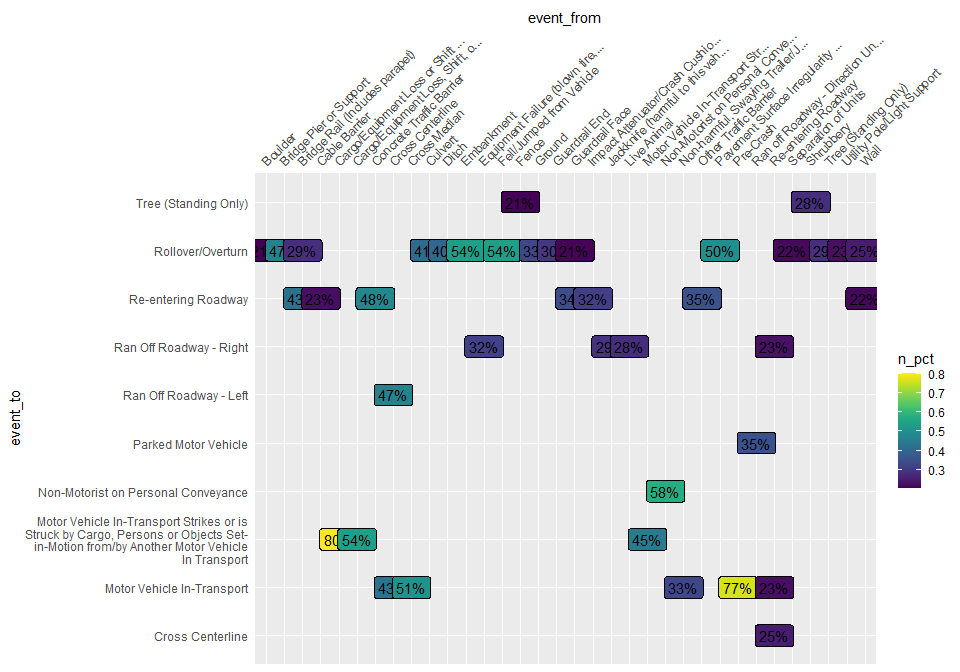# Crash Sequences

This vignette explores the vsoe (Vehicle Sequence of Events) data to visualize crash sequence patterns.

vsoe is one of three event-based data files, the others being cevent and vevent. According to the CRSS Analytical User’s Manual, vevent “has the same data elements as the cevent data file” plus “a data element that records the sequential event number for each vehicle,” and the vsoe file “has a subset of the data elements contained in the Vevent data file (it is a simplified vevent data file)” (p. 16). rfars therefore omits cevent and vevent.

First we get one year of data, and filter to the southern region for simplicity.

mydata <- rfars::get_gescrss(years=2021, regions = "s")
#>
#> ── Column specification ────────────────────────────────────────────────────────
#> cols(
#>   .default = col_character(),
#>   year = col_double(),
#>   psu = col_double(),
#>   psustrat = col_double(),
#>   casenum = col_double(),
#>   weight = col_double(),
#>   id = col_double(),
#>   veh_no = col_double(),
#>   per_no = col_double(),
#>   minute = col_double(),
#>   mod_year = col_logical(),
#>   model = col_double(),
#>   peds = col_double(),
#>   permvit = col_double(),
#>   pernotmvit = col_double(),
#>   psu_var = col_double(),
#>   pvh_invl = col_double(),
#>   str_veh = col_double(),
#>   ve_forms = col_double(),
#>   ve_total = col_double()
#> )
#> ℹ Use spec() for the full column specifications.

my_events <- mydata\$events

The Vsoe data is stored in the events tibble of the object returned by get_gescrss(). Here we see the top 10 individual events:

my_events %>%
group_by(soe) %>% summarize(n=n()) %>%
arrange(desc(n)) %>%
slice(1:10)
#> # A tibble: 10 × 2
#>    soe                            n
#>    <chr>                      <int>
#>  1 Motor Vehicle In-Transport 82664
#>  2 Ran Off Roadway - Right     8191
#>  3 Parked Motor Vehicle        5914
#>  4 Ran Off Roadway - Left      5529
#>  5 Rollover/Overturn           3460
#>  6 Pedestrian                  2917
#>  7 Cross Centerline            2638
#>  8 Tree (Standing Only)        2076
#>  9 Live Animal                 1869
#> 10 Pedalcyclist                1822

We can also see the top 10 most common sequences:

my_events %>%
select(-aoi) %>%
pivot_wider(names_from = "veventnum", values_from = "soe", values_fill = "x",
names_prefix = "event") %>%
select(starts_with("event")) %>%
group_by_all() %>%
summarize(n=n(), .groups = "drop") %>%
arrange(desc(n)) %>%
slice(1:10) %>%
select(event1, event2, n)
#> # A tibble: 10 × 3
#>    event1                     event2                         n
#>    <chr>                      <chr>                      <int>
#>  1 Motor Vehicle In-Transport x                          67806
#>  2 Motor Vehicle In-Transport Motor Vehicle In-Transport  4106
#>  3 Parked Motor Vehicle       x                           2996
#>  4 Pedestrian                 x                           2291
#>  5 Live Animal                x                           1772
#>  6 Pedalcyclist               x                           1703
#>  7 Ran Off Roadway - Right    Parked Motor Vehicle         848
#>  8 Cross Centerline           Motor Vehicle In-Transport   752
#>  9 Ran Off Roadway - Right    Utility Pole/Light Support   418
#> 10 Rollover/Overturn          x                            404

Below we consider all state transitions - the transition from one event to the next in the sequence. For example, the sequence A-B-C-D has three transitions: A to B, B to C, and C to D. The graph below shows a subset of the more common transitions in the crash sequences. It is interpreted as follows: the event listed on the x-axis (top) was followed by the event listed on the y-axis. The percentage shown at the graphical intersection represents the percentage of transitions from one event (x) to another event (y). For example, ‘Fence’ was followed by ‘Tree (Standing Only)’ in 21% of sequences. Note that we have added a state labelled ‘Pre-Crash’ to help account for sequences with just one event. Another notable takeaway is the large number of events that precede ’Rollover/Overturn.

my_events %>%
group_by(year, casenum, veh_no) %>%
dplyr::rename(event_to = soe) %>%
mutate(event_from = data.table::shift(event_to, fill = "Pre-Crash")) %>%
select(event_from, event_to) %>%
group_by(event_from, event_to) %>% summarize(n=n()) %>%
group_by(event_from) %>% mutate(n_from = sum(n)) %>%
mutate(n_pct = n/n_from) %>%
filter(n_pct>.2, n>5) %>%
mutate(
event_from = ifelse(nchar(event_from)>30, paste0(stringr::str_sub(event_from, 1, 30), "..."), event_from),
#event_to   = paste0(stringr::str_sub(event_to, 1, 30), "..."),
event_to = stringr::str_wrap(event_to, 40)
) %>%
filter(event_from != event_to) %>%

ggplot(aes(x=event_from, y=event_to, fill=n_pct, label=scales::percent(n_pct, accuracy = 1))) +
viridis::scale_fill_viridis() +
geom_label() +
scale_x_discrete(position = "top") +
theme(
axis.text.x.top = element_text(angle=45, hjust=0),
axis.ticks = element_blank(),
#axis.text.x.bottom = element_text(angle=270, hjust = 0, vjust=.5),
#legend.position = "none"
)
#> Adding missing grouping variables: year, casenum, veh_no
#> summarise() has grouped output by 'event_from'. You can override using the
#> .groups argument.